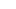# Using Offset Function in Excel

February 15, 2019

Offset returns a reference to a range that is a specified number of rows and columns from a cell or range of cells. The reference returned can be a single cell or a range of cells.

Let's first see the formula in detail and then try its practical usage. Offset function has the following parameters.

=offset(reference, rows, columns, [height], [width])

Let's see each parameter in detail

Reference: This is a range or a cell which would be the starting point.

Row: This is the number of rows up/down away from the starting point.

Column: This is the number of columns left/right away from the starting point.

[height]: The number of rows to be considered.

[Width]: The number of columns to be considered (generally it is 1)

Now let's use the below table to understand how to use basic offset function. In the table below we are sure that our data starts in cell A1.

I want to have the salary of 3rd employee. In this case its 'Sam' and his salary is 3000.

I want the result in cell A11.

Now this is a very basic use of offset and one can argue that a direct reference would work without using 'offset' something like this in cell A11: =D4

As said, this is just to understand how Offset works and we can see its actual use in couple of more examples which would follow.

So to get the salary of 3rd employee where data starts from A1, I would use offset function and tell it to start in Cell A1 (reference), move 3 rows down (rows), move 3 columns right (columns), only 1 cell to be considered so the next 2 parameters are 1 (height) and 1 (width).

If the formula is written in A11 it would be something like the below.

This would result in the answer as 3000

Now let's expand the requirement. We need the sum of salaries of Sam and Carl who are 3 rows and 4 rows away from cell A1.

So to get the sum using offset the parameters inside the sum function would be Cell A1 (reference), move 3 rows down (rows), move 3 columns right (columns), 2 cells to be considered so the height is 2 and width is 1.

If the formula is written in cell A11 it would be something like the below.

This would result in the answer as 5000

Taking a step forward in the next post we will see how to use offset function to get YTD (Year to Date) sum or running totals.

###### Featured PostsExcel Demo Video

November 14, 2014

1/2